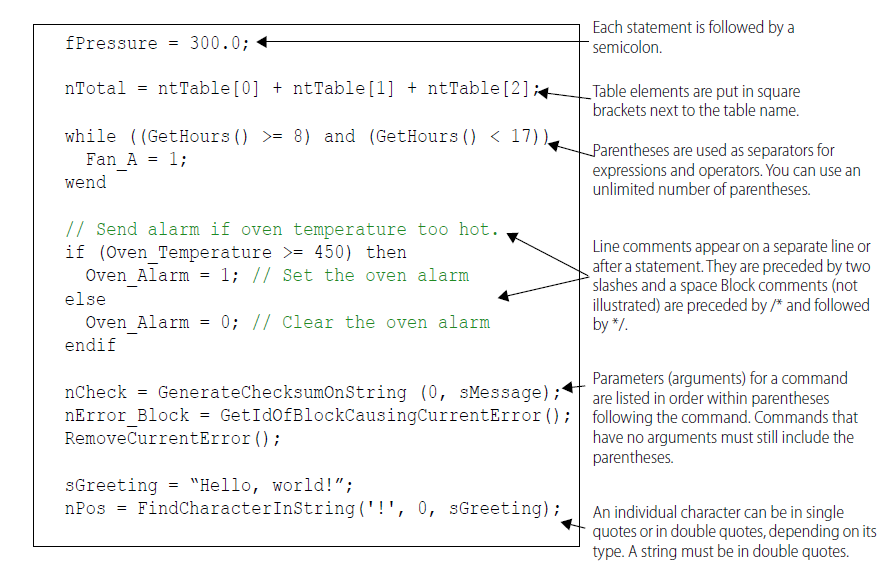## OptoScript Syntax

Let’s take a look at this sample OptoScript code to demonstrate the syntax.Arguments for a command are listed in the parentheses following the command, as seen here:

SetDownTimerPresetValue        (60.0, Minute_Timer)

command                               (argument 1, argument 2)

Arguments are listed in order beginning with argument 1. To find out the arguments for any command, see the PAC Control Command Reference or online Command Help.

### Types of Commands

There are two types of OptoScript commands: procedure commands and function commands.

Procedure commands accomplish an action and return no value. Here are some examples:

RemoveCurrentError();
DelayMsec(100);

Function commands return a value from their action, so the value can be placed somewhere. In the following examples, the value is placed in the variable at the beginning of the statement (the variable to the left of =):

nMonth = GetMonth();
fSquare_Root = SquareRoot(99);
nPosition = FindCharacterInString('S',0, sName);

When you compare these examples to the identical commands in standard PAC Control, you’ll notice that the returned value for the standard command is an argument. In OptoScript, the returned value is not an argument, reducing the number of arguments by one.

In the first example, the standard command Get Month has one argument, which is where the result is placed. The OptoScript command equivalent, GetMonth, has no arguments and places the result in the variable.

In most cases you'll use the value a function command returns by placing it in a variable, a control structure, or a mathematical expression. Occasionally, however, you may not need to use the result. For example, the command StartChart returns a status. If you don't need to track the status, you can ignore it by not placing the result anywhere, for example:

StartChart(Chart_Status);

For a complete explanation of the syntax in OptoScript, refer to the PAC Control User's Guide, Appendix E: OptoScript Language Reference.

#### Summary

Learn from an example that demonstrates the syntax for OptoScript, followed by a brief discussion about the syntax for procedure and function commands.